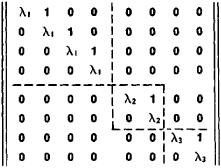# Jordan Matrix

(redirected from Canonical box matrix)

## Jordan matrix

[zhȯr′dän‚mā·triks]
(mathematics)
A matrix whose elements are equal and nonzero on the principal diagonal, equal to 1 on the diagonal immediately above, and equal to 0 everywhere else.
McGraw-Hill Dictionary of Scientific & Technical Terms, 6E, Copyright © 2003 by The McGraw-Hill Companies, Inc.
The following article is from The Great Soviet Encyclopedia (1979). It might be outdated or ideologically biased.

## Jordan Matrix

(also Jordan form of a matrix, or simple classical matrix). With every square matrix A = ǀǀaikǀǀn1 there is associated a class of similar matrices. This class of matrices always includes a matrix of special normal (canonical) Jordan form, named after M. E. C. Jordan. The Jordan form of a certain eighth-order matrix is given in Figure 1.Figure 1

Special square blocks, enclosed by broken lines in Figure 1, lie on the principal diagonal. All elements of the matrix that lie outside these blocks are equal to zero. The (complex) numbers forming the principal diagonal of a block are the same; for example, in the first block they are all equal to λ1, and in the second to λ2. The elements of the diagonal immediately above the principal diagonal equal to unity. All other elements in the diagonal blocks are equal to zero. The matrix in Figure 1 contains three diagonal blocks, of which the first is of order 4, and the second and third of order 2. In the general case, the number of blocks and their orders are arbitrary. It is also possible for some of the numbers λ1, λ2, … to be equal. The initial matrix A in this example has the elementary divisors (λ – λ1)4, (λ – λ2)2, and (λ – λ3)2. A Jordan matrix is uniquely determined by its elementary divisors.

If the matrix A has the Jordan form I, then there exists a nonsingular matrix T such that A = TIT–1. Replacement of matrix A by the similar Jordan matrix I is called a reduction of matrix A to normal Jordan form.

To get an idea of the uses of the Jordan form of a matrix, we consider a system of linear differential equations with constant coefficients:

dx1/dt = a11x1 + a12x2 + … + a1nxn

dx2/dt = a21x1 + a22x2 + … + a2nxn

dxn/dt = an1x1 + an2x2 + … + annxn

In matrix notation we have

(1) dx/dt = Ax

Let us introduce new unknown functions y1, y2, …, yn by using a nonsingular matrix T = ǀǀtikǀǀn1 where the tik are numbers (i, k = 1, 2, …, n):

x1 = t11y1 + t12y2 + … + t1nyn

x1 = t21y1 + t22y2 + … + t2nyn

xn = tn1y1 + tn2y2 + … + tnnyn

or in matrix notation

x = Ty

Substituting this expression for x in (1), we obtain

(2) dy/dt = Iy

where matrix I is related to matrix A by the equation

A = TIT–1

The matrix T is usually selected in such a way that matrix A is a Jordan matrix. In this case, the system of equations (2) is much simpler than system (1). For example, if matrix A = ǀǀaikǀǀ81 (n = 8) has the Jordan form given in Figure 1, then system (2) will have the form

dy1/dt = λ1y1 + y2dy5/dt = λ2y5 + y6

dy2/dt = λ1y2 + y3dy6/dt = λ2y6

dy3/dt = λ1y3 + y4dy7/dt = λ3y7 + y8

dy4/dt = λ1y4dy8/dt = λ3y8

Integration of this system reduces to a number of integrations of single differential equations.

### REFERENCES

See references under .
The Great Soviet Encyclopedia, 3rd Edition (1970-1979). © 2010 The Gale Group, Inc. All rights reserved.
Site: Follow: Share:
Open / Close